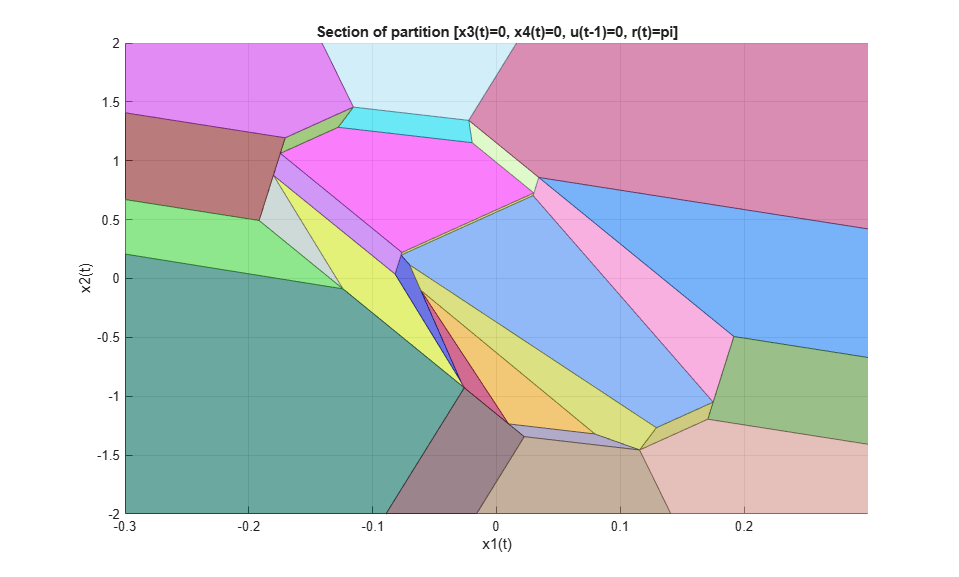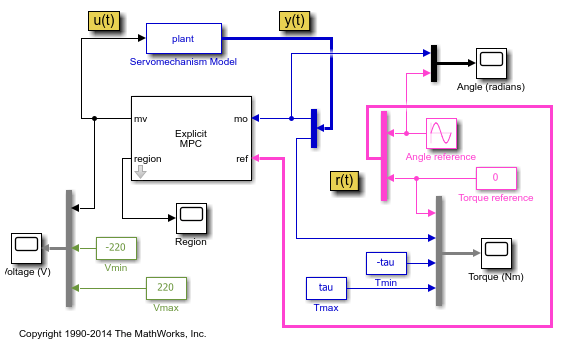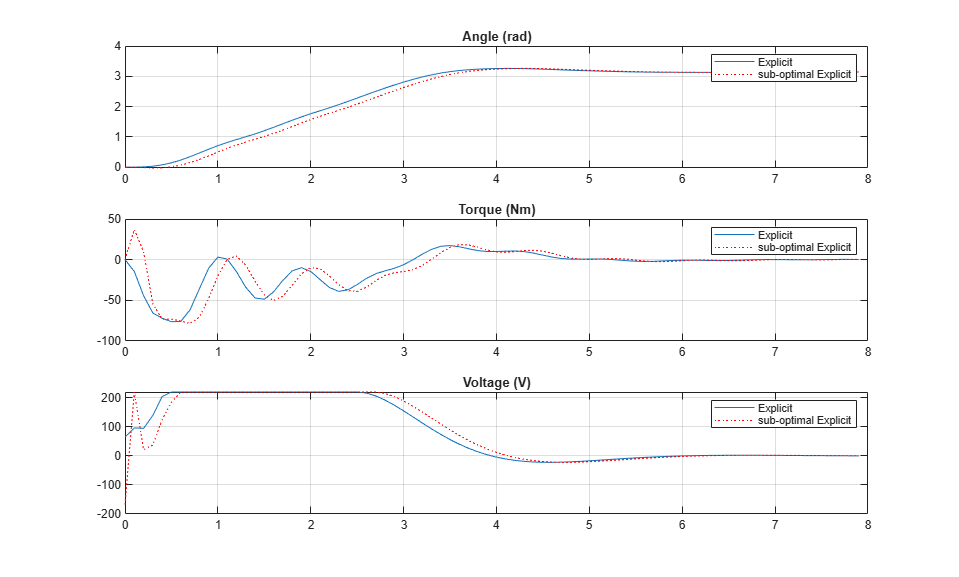Documentation

### This is machine translation

Mouseover text to see original. Click the button below to return to the English version of the page.

## Explicit MPC Control of DC Servomotor with Constraint on Unmeasured Output

This example shows how to use Explicit MPC to control DC servomechanism under voltage and shaft torque constraints.

Reference

 A. Bemporad and E. Mosca, ''Fulfilling hard constraints in uncertain linear systems by reference managing,'' Automatica, vol. 34, no. 4, pp. 451-461, 1998.

### Define DC-Servo Motor Model

The linear open-loop dynamic model is defined in "plant". Variable "tau" is the maximum admissible torque to be used as an output constraint.

```[plant, tau] = mpcmotormodel; ```

### Design MPC Controller

Specify input and output signal types for the MPC controller. The second output, torque, is unmeasurable.

```plant = setmpcsignals(plant,'MV',1,'MO',1,'UO',2); ```

MV Constraints

The manipulated variable is constrained between +/- 220 volts. Since the plant inputs and outputs are of different orders of magnitude, you also use scale factors to facilitate MPC tuning. Typical choices of scale factor are the upper/lower limit or the operating range.

```MV = struct('Min',-220,'Max',220,'ScaleFactor',440); ```

OV Constraints

Torque constraints are only imposed during the first three prediction steps to limit the complexity of the explicit MPC design.

```OV = struct('Min',{Inf, [-tau;-tau;-tau;-Inf]},'Max',{Inf, [tau;tau;tau;Inf]},'ScaleFactor',{2*pi, 2*tau}); ```

Weights

The control task is to get zero tracking offset for the angular position. Since you only have one manipulated variable, the shaft torque is allowed to float within its constraint by setting its weight to zero.

```Weights = struct('MV',0,'MVRate',0.1,'OV',[0.1 0]); ```

Construct MPC controller

Create an MPC controller with plant model, sample time and horizons.

```Ts = 0.1; % Sampling time p = 10; % Prediction horizon m = 2; % Control horizon mpcobj = mpc(plant,Ts,p,m,Weights,MV,OV); ```

### Generate Explicit MPC Controller

Explicit MPC executes the equivalent explicit piecewise affine version of the MPC control law defined by the traditional MPC. To generate an Explicit MPC from a traditional MPC, you must specify the range for each controller state, reference signal, manipulated variable and measured disturbance so that the multi-parametric quadratic programming problem is solved in the parameter sets defined by these ranges.

Obtain a range structure for initialization

Use `generateExplicitRange` command to obtain a range structure where you can specify the range for each parameter afterwards.

```range = generateExplicitRange(mpcobj); ```
```-->Converting model to discrete time. Assuming no disturbance added to measured output channel #1. -->The "Model.Noise" property of the "mpc" object is empty. Assuming white noise on each measured output channel. ```

Specify ranges for controller states

MPC controller states include states from plant model, disturbance model and noise model in that order. Setting the range of a state variable is sometimes difficult when the state does not correspond to a physical parameter. In that case, multiple runs of open-loop plant simulation with typical reference and disturbance signals are recommended in order to collect data that reflect the ranges of states.

```range.State.Min(:) = -1000; range.State.Max(:) = 1000; ```

Specify ranges for reference signals

Usually you know the practical range of the reference signals being used at the nominal operating point in the plant. The ranges used to generate Explicit MPC must be at least as large as the practical range. Note that the range for torque reference is fixed at 0 because it has zero weight.

```range.Reference.Min = [-5;0]; range.Reference.Max = [5;0]; ```

Specify ranges for manipulated variables

If manipulated variables are constrained, the ranges used to generate Explicit MPC must be at least as large as these limits.

```range.ManipulatedVariable.Min = MV.Min - 1; range.ManipulatedVariable.Max = MV.Max + 1; ```

Construct the Explicit MPC controller

Use `generateExplicitMPC` command to obtain the Explicit MPC controller with the parameter ranges previously specified.

```mpcobjExplicit = generateExplicitMPC(mpcobj, range); display(mpcobjExplicit); ```
``` Regions found / unexplored: 75/ 0 Explicit MPC Controller --------------------------------------------- Controller sample time: 0.1 (seconds) Polyhedral regions: 75 Number of parameters: 6 Is solution simplified: No State Estimation: Default Kalman gain --------------------------------------------- Type 'mpcobjExplicit.MPC' for the original implicit MPC design. Type 'mpcobjExplicit.Range' for the valid range of parameters. Type 'mpcobjExplicit.OptimizationOptions' for the options used in multi-parametric QP computation. Type 'mpcobjExplicit.PiecewiseAffineSolution' for regions and gain in each solution. ```

### Plot Piecewise Affine Partition

You can review any 2-D section of the piecewise affine partition defined by the Explicit MPC control law.

Obtain a plot parameter structure for initialization

Use `generatePlotParameters` command to obtain a parameter structure where you can specify which 2-D section to plot afterwards.

```params = generatePlotParameters(mpcobjExplicit); ```

Specify parameters for a 2-D plot

In this example, you plot the 1th state variable vs. the 2nd state variable. All the other parameters must be fixed at a value within its range.

Fix other state variables

```params.State.Index = [3 4]; params.State.Value = [0 0]; ```

Fix reference signals

```params.Reference.Index = [1 2]; params.Reference.Value = [pi 0]; ```

Fix manipulated variables

```params.ManipulatedVariable.Index = 1; params.ManipulatedVariable.Value = 0; ```

Plot the 2-D section

Use `plotSection` command to plot the 2-D section defined previously.

```plotSection(mpcobjExplicit, params); axis([-.3 .3 -2 2]); grid title('Section of partition [x3(t)=0, x4(t)=0, u(t-1)=0, r(t)=pi]') xlabel('x1(t)'); ylabel('x2(t)'); ```### Simulate Using SIM Command

Compare closed-loop simulation between traditional MPC (as referred as Implicit MPC) and Explicit MPC

```Tstop = 8; % seconds Tf = round(Tstop/Ts); % simulation iterations r = [pi 0]; % reference signal [y1,t1,u1] = sim(mpcobj,Tf,r); % simulation with traditional MPC [y2,t2,u2] = sim(mpcobjExplicit,Tf,r); % simulation with Explicit MPC ```
```-->Converting model to discrete time. Assuming no disturbance added to measured output channel #1. -->The "Model.Noise" property of the "mpc" object is empty. Assuming white noise on each measured output channel. ```

The simulation results are identical.

```fprintf('SIM command: Difference between QP-based and Explicit MPC trajectories = %g\n',norm(u2-u1)+norm(y2-y1)); ```
```SIM command: Difference between QP-based and Explicit MPC trajectories = 8.68909e-12 ```

To run this example, Simulink® is required.

```if ~mpcchecktoolboxinstalled('simulink') disp('Simulink(R) is required to run this example.') return end ```

Simulate closed-loop control of the linear plant model in Simulink, using the Explicit MPC Controller block. Controller "mpcobjExplicit" is specified in the block dialog.

```mdl = 'empc_motor'; open_system(mdl) sim(mdl); ```The closed-loop response is identical to the traditional MPC controller designed in the "mpcmotor" example.

### Control Using Sub-optimal Explicit MPC

To reduce the memory footprint, you can use `simplify` command to reduce the number of piecewise affine solution regions. For example, you can remove regions whose Chebychev radius is smaller than .08. However, the price you pay is that the controller performance now becomes sub-optimal.

Use `simplify` command to generate Explicit MPC with sub-optimal solutions.

```mpcobjExplicitSimplified = simplify(mpcobjExplicit, 'radius', 0.08); disp(mpcobjExplicitSimplified); ```
``` Regions to analyze: 75/ 75 --> 37 regions deleted. explicitMPC with properties: MPC: [1x1 mpc] Range: [1x1 struct] OptimizationOptions: [1x1 struct] PiecewiseAffineSolution: [1x38 struct] IsSimplified: 1 ```

The number of piecewise affine regions has been reduced.

Compare closed-loop simulation between sub-optimal Explicit MPC and Explicit MPC.

```[y3,t3,u3] = sim(mpcobjExplicitSimplified, Tf, r); ```
```-->Converting model to discrete time. Assuming no disturbance added to measured output channel #1. -->The "Model.Noise" property of the "mpc" object is empty. Assuming white noise on each measured output channel. ```

The simulation results are not the same.

```fprintf('SIM command: Difference between exact and suboptimal MPC trajectories = %g\n',norm(u3-u2)+norm(y3-y2)); ```
```SIM command: Difference between exact and suboptimal MPC trajectories = 439.399 ```

Plot results.

```figure; subplot(3,1,1) plot(t1,y1(:,1),t3,y3(:,1),'o'); grid title('Angle (rad)') legend('Explicit','sub-optimal Explicit') subplot(3,1,2) plot(t1,y1(:,2),t3,y3(:,2),'o'); grid title('Torque (Nm)') legend('Explicit','sub-optimal Explicit') subplot(3,1,3) plot(t1,u1,t3,u3,'o'); grid title('Voltage (V)') legend('Explicit','sub-optimal Explicit') ```The simulation result with the sub-optimal Explicit MPC is slightly worse.

```bdclose(mdl) ```